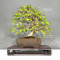##### The Japanese Bonsai specialist
Direct order Contact Help / Services Newsletter# Carpinus turczaninowii ref :13100172

Carpinus turczaninowiiref. : 11929

Product unavailable

###### Description

Height of the only tree: 360 mm.
Width of the branches: 460 mm.

Dimensions of the non-enameled pot: 270*200*95 mm. Moyogi style. Deciduous foliage, green in spring and in summer, fast growth. Splendid and very natural hornbeam tree.

The Korean and Japanese hornbeam trees not only present a hanging catkin flowering but also beautiful autumn colors. No strong wire marks or cutting wounds. It was repoted in Akadama soil in 2022 spring. The turczaninowii carpinus has the particularity to have a superb white bark that becomes veined as it gets older like our beech trees. Photographed in May 2023. Shelf not included.

#turczaninowii 4.3 #deciduous 3.9 #carpinus 3.8 #hornbeam 2.8 #spring 2.6 #bonsai 2.6 #trees 2.5 #tree 2.4 #only 2.4 #particularity 2.3

Formule
(( ROUND((CHAR_LENGTH(b.article_nom)-CHAR_LENGTH(REPLACE(b.article_nom, 'turczaninowii', '')))/LENGTH('turczaninowii')) + ROUND((CHAR_LENGTH(b.article_description)-CHAR_LENGTH(REPLACE(b.article_description, 'turczaninowii', '')))/LENGTH('turczaninowii')) ) * 4.3) + (( ROUND((CHAR_LENGTH(b.article_nom)-CHAR_LENGTH(REPLACE(b.article_nom, 'carpinus', '')))/LENGTH('carpinus')) + ROUND((CHAR_LENGTH(b.article_description)-CHAR_LENGTH(REPLACE(b.article_description, 'carpinus', '')))/LENGTH('carpinus')) ) * 3.8) + (( ROUND((CHAR_LENGTH(b.article_nom)-CHAR_LENGTH(REPLACE(b.article_nom, 'hornbeam', '')))/LENGTH('hornbeam')) + ROUND((CHAR_LENGTH(b.article_description)-CHAR_LENGTH(REPLACE(b.article_description, 'hornbeam', '')))/LENGTH('hornbeam')) ) * 2.8) + (( ROUND((CHAR_LENGTH(b.article_nom)-CHAR_LENGTH(REPLACE(b.article_nom, 'spring', '')))/LENGTH('spring')) + ROUND((CHAR_LENGTH(b.article_description)-CHAR_LENGTH(REPLACE(b.article_description, 'spring', '')))/LENGTH('spring')) ) * 2.6) + (( ROUND((CHAR_LENGTH(b.article_nom)-CHAR_LENGTH(REPLACE(b.article_nom, 'trees', '')))/LENGTH('trees')) + ROUND((CHAR_LENGTH(b.article_description)-CHAR_LENGTH(REPLACE(b.article_description, 'trees', '')))/LENGTH('trees')) ) * 2.5) + (( ROUND((CHAR_LENGTH(b.article_nom)-CHAR_LENGTH(REPLACE(b.article_nom, 'tree', '')))/LENGTH('tree')) + ROUND((CHAR_LENGTH(b.article_description)-CHAR_LENGTH(REPLACE(b.article_description, 'tree', '')))/LENGTH('tree')) ) * 2.4) + (( ROUND((CHAR_LENGTH(b.article_nom)-CHAR_LENGTH(REPLACE(b.article_nom, 'only', '')))/LENGTH('only')) + ROUND((CHAR_LENGTH(b.article_description)-CHAR_LENGTH(REPLACE(b.article_description, 'only', '')))/LENGTH('only')) ) * 2.4) + (( ROUND((CHAR_LENGTH(b.article_nom)-CHAR_LENGTH(REPLACE(b.article_nom, 'particularity', '')))/LENGTH('particularity')) + ROUND((CHAR_LENGTH(b.article_description)-CHAR_LENGTH(REPLACE(b.article_description, 'particularity', '')))/LENGTH('particularity')) ) * 2.3) + (( ROUND((CHAR_LENGTH(b.article_nom)-CHAR_LENGTH(REPLACE(b.article_nom, 'photographed', '')))/LENGTH('photographed')) + ROUND((CHAR_LENGTH(b.article_description)-CHAR_LENGTH(REPLACE(b.article_description, 'photographed', '')))/LENGTH('photographed')) ) * 2.2) + (( ROUND((CHAR_LENGTH(b.article_nom)-CHAR_LENGTH(REPLACE(b.article_nom, 'dimensions', '')))/LENGTH('dimensions')) + ROUND((CHAR_LENGTH(b.article_description)-CHAR_LENGTH(REPLACE(b.article_description, 'dimensions', '')))/LENGTH('dimensions')) ) * 2)

## Secure payment## Delivery

Our logistic partners :04 74 55 23 48
Pépinière MAILLOT-BONSAÏ
Le Bois Frazy
01990 RELEVANT - FRANCE
on appointment